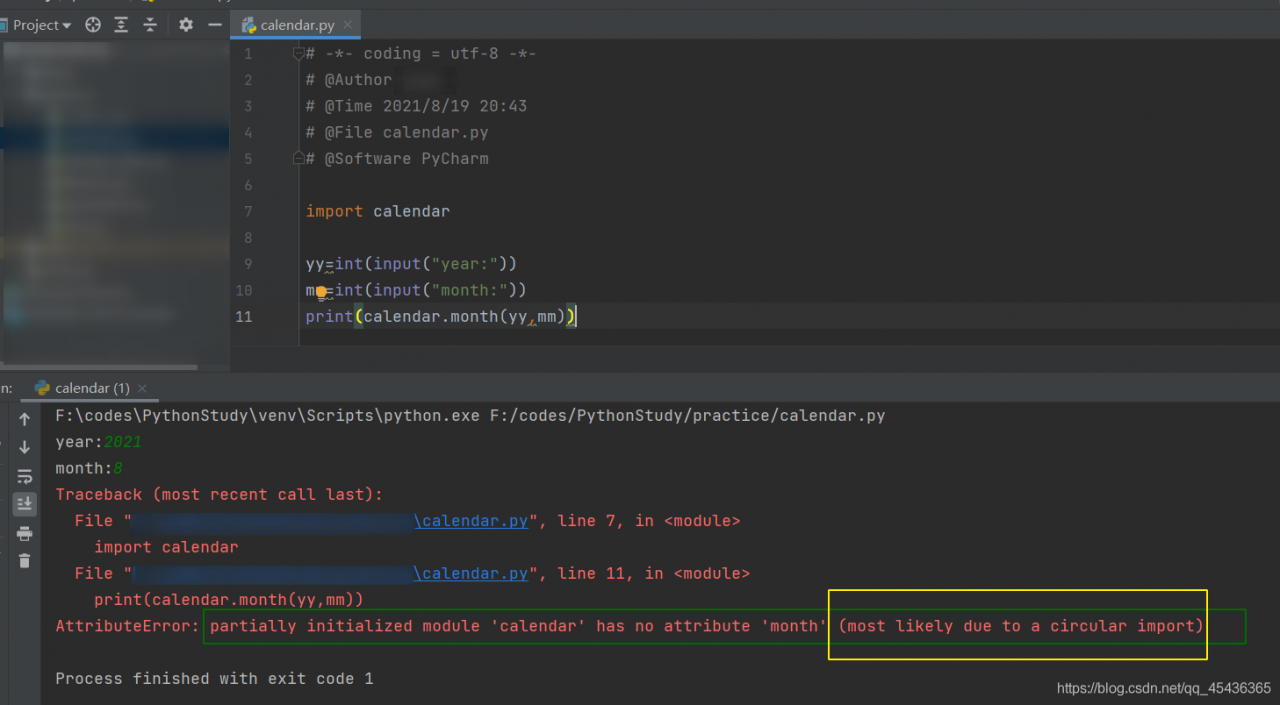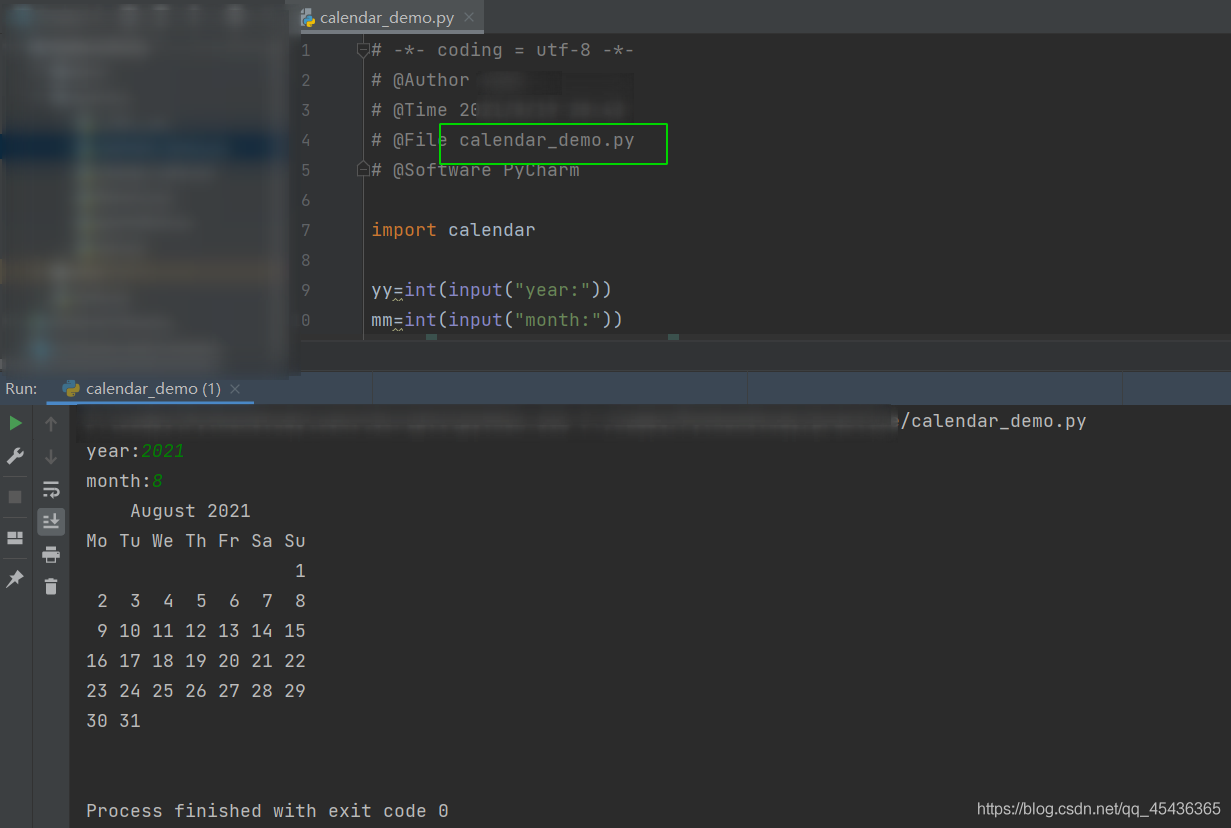# Python calendar.month() Error [How to Solve]

When calling calendar.month today, an error is reported. The
code is as follows

``````import calendar

yy=int(input("year:"))
mm=int(input("month:"))
print(calendar.month(yy,mm))
``````

The error report is shown in the figure belowlook at the yellow part circled in the figure. Circular import may be circular. You can see that the program file name is calendar.py, which is consistent with the introduced calendar, so it causes an error.

Solution
just modify the file name, which cannot have the same name as the imported package